# Program for Picard’s iterative method | Computational Mathematics

The Picard’s method is an iterative method and is primarily used for approximating solutions to differential equations.

This method of solving a differential equation approximately is one of successive approximation; that is, it is an iterative method in which the numerical results become more and more accurate, the more times it is used.

The Picard’s iterative method gives a sequence of approximations Y1(x), Y2(x), …Yk(x) to the solution of differential equations such that the nth approximation is obtained from one or more previous approximations.

The Picard’s iterative series is relatively easy to implement and the solutions obtained through this numerical analysis are generally power series.

Picard’s iteration method formula:

Steps involved:

• Step 1: An approximate value of y (taken, at first, to be a constant) is substituted into the right hand side of the differential equation:
dy/dx= f(x, y).
• Step 2: The equation is then integrated with respect to x giving y in terms of x as a second approximation, into which given numerical values are substituted and the result rounded off to an assigned number of decimal places or significant figures.
• Step 3: The iterative process is continued until two consecutive numerical solutions are the same when rounded off to the required number of decimal places.

Picard’s iteration example:
Given that: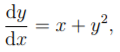and that y = 0 when x = 0, determine the value of y when x = 0.3, correct to four places of decimals.

Solution:
We may proceed as follows: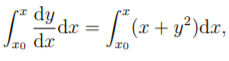where x0 = 0. Hence: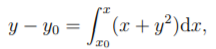where y0 = 0. which becomes: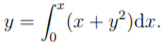• First Iteration:
We do not know y in terms of x yet, so we replace y by the constant value y0 in the function to be integrated.

The result of the first iteration is thus given, at x = 0.3, by: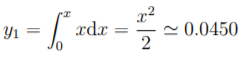• Second Iteration:
Now, we use: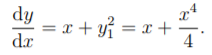Therefore,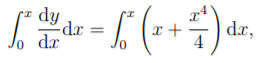which gives: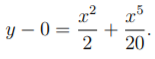The result of the second iteration is thus given by: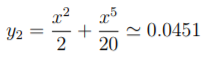at x=0.3.

• Third Iteration:
Now we use: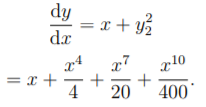Therefore,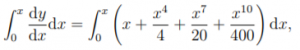which gives: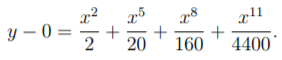The result of the third iteration is thus given by: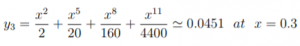at x = 0.3.

• Hence, y = 0.0451, correct upto four decimal places, at x = 0.3.

Program for Picard’s iterative method:

 `// C program for Picard's iterative method ` ` `  `#include ` `#include ` ` `  `// required macros defined below: ` `#define Y1(x) (1 + (x) + pow(x, 2) / 2) ` `#define Y2(x) (1 + (x) + pow(x, 2) / 2 + pow(x, 3) / 3 + pow(x, 4) / 8) ` `#define Y3(x) (1 + (x) + pow(x, 2) / 2 + pow(x, 3) / 3 + pow(x, 4) / 8 + pow(x, 5) / 15 + pow(x, 6) / 48) ` ` `  `int` `main() ` `{ ` `    ``double` `start_value = 0, end_value = 3, ` `           ``allowed_error = 0.4, temp; ` `    ``double` `y1, y2, y3; ` `    ``int` `count; ` ` `  `    ``for` `(temp = start_value, count = 0; ` `         ``temp <= end_value; ` `         ``temp = temp + allowed_error, count++) { ` ` `  `        ``y1[count] = Y1(temp); ` `        ``y2[count] = Y2(temp); ` `        ``y3[count] = Y3(temp); ` `    ``} ` ` `  `    ``printf``(``"\nX\n"``); ` `    ``for` `(temp = start_value; ` `         ``temp <= end_value; ` `         ``temp = temp + allowed_error) { ` ` `  `        ``// considering all values ` `        ``// upto 4 decimal places. ` `        ``printf``(``"%.4lf "``, temp); ` `    ``} ` ` `  `    ``printf``(``"\n\nY(1)\n"``); ` `    ``for` `(temp = start_value, count = 0; ` `         ``temp <= end_value; ` `         ``temp = temp + allowed_error, count++) { ` ` `  `        ``printf``(``"%.4lf "``, y1[count]); ` `    ``} ` ` `  `    ``printf``(``"\n\nY(2)\n"``); ` `    ``for` `(temp = start_value, count = 0; ` `         ``temp <= end_value; ` `         ``temp = temp + allowed_error, count++) { ` ` `  `        ``printf``(``"%.4lf "``, y2[count]); ` `    ``} ` ` `  `    ``printf``(``"\n\nY(3)\n"``); ` `    ``for` `(temp = start_value, count = 0; ` `         ``temp <= end_value; ` `         ``temp = temp + allowed_error, count++) { ` ` `  `        ``printf``(``"%.4lf "``, y3[count]); ` `    ``} ` `    ``return` `0; ` `} `

Output:

```X
0.0000 0.4000 0.8000 1.2000 1.6000 2.0000 2.4000 2.8000

Y(1)
1.0000 1.4800 2.1200 2.9200 3.8800 5.0000 6.2800 7.7200

Y(2)
1.0000 1.5045 2.3419 3.7552 6.0645 9.6667 15.0352 22.7205

Y(3)
1.0000 1.5053 2.3692 3.9833 7.1131 13.1333 24.3249 44.2335
```

GeeksforGeeks has prepared a complete interview preparation course with premium videos, theory, practice problems, TA support and many more features. Please refer Placement 100 for details

My Personal Notes arrow_drop_upCheck out this Author's contributed articles.

If you like GeeksforGeeks and would like to contribute, you can also write an article using contribute.geeksforgeeks.org or mail your article to contribute@geeksforgeeks.org. See your article appearing on the GeeksforGeeks main page and help other Geeks.

Please Improve this article if you find anything incorrect by clicking on the "Improve Article" button below.